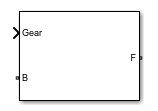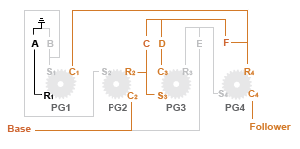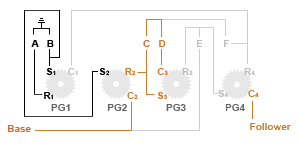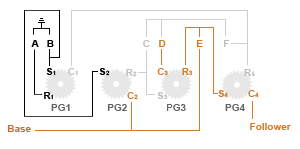# 10-Speed

Clutch schedule for a 10-speed transmission

• Library:
• Simscape / Driveline / Transmissions

•## Description

The 10-Speed transmission block consists of four planetary gear sets and six clutches. The follower shaft connects to the planet gear carrier of the fourth planetary gear. Four of the clutches determine the power flow path for the base shaft. The other two clutches serve as brakes, grounding various gears of the planetary sets to the transmission housing.

This diagram shows a 10-speed transmission. The labels for the gear components are superimposed on the input and output gears. The table lists the gear and clutch components that are labeled in the diagram. Power elements are shown in orange. Braking elements are shown in black.LabelComponent
PG1–PG4Planetary gears, 1–4
Rx Ring gear of planetary gear x
CxPlanet gear carrier of planetary gear x
SxSun gear of planetary gear x
C, D, E, FClutches that control the power flow path
A, BBraking clutches

### Drive Ratios, Clutch Schedule, and Power Flow

The drive ratio between the transmission input and output shafts follows from the elementary gear ratios specified for the gear blocks. The elementary gear ratios are

`${g}_{x}=\frac{{N}_{{R}_{x}}}{{N}_{{S}_{x}}},$`

where:

• NRx is the number of teeth in the planetary ring gear x, where x = 1, 2, 3, and 4.

• NSx is the number of teeth in the planetary sun gear x, where x = 1, 2, 3, and 4

The table shows the clutch schedule, drive-ratio expressions, drive-ratio default values, and the power-flow diagrams for each gear of the 10-Speed block. The schedule and gear ratios are based on the manufacturer data for a 10R80 10-speed automatic transmission.

The letters in the clutch schedule columns denote the brakes and clutches. A value of `1` denotes a locked state and a value of `0` an unlocked state. The clutch schedule generates these signals based on the Gear port input signal. The signals are scaled through a Gain block and used as actuation inputs in the clutch blocks.

The power-flow diagrams show the power flow paths between input and output shafts for each gear setting. Power flow is shown in orange. Connections to the transmission housing (a mechanical ground) are shown in black.

GearClutch ScheduleDrive Ratio EquationDefault RatioPower Flow
ABCDEF
10011101$\frac{{g}_{2}}{1+{g}_{2}}$0.649011011$\frac{{g}_{2}\left(1+{g}_{4}\right)}{\left({g}_{2}\left(1+{g}_{4}\right)\right)+{g}_{4}}$0.698010111$\frac{{g}_{2}\left(1+{g}_{3}\right)\left(1+{g}_{4}\right)}{\left({g}_{2}\left(1+{g}_{3}\right)\left(1+g4\right)\right)+{g}_{4}}$0.857001111$1$16100111$\frac{\left(1+{g}_{4}\right)\left(1+{g}_{1}+{g}_{2}\left(1+{g}_{3}\right)\right)}{\left(1+{g}_{4}\right)\left(1+{g}_{2}\left(1+{g}_{3}\right)\right)+{g}_{1}}$1.275101011$\frac{\left(1+{g}_{1}+{g}_{2}\right)\left(1+{g}_{4}\right)}{\left(1+{g}_{2}\right)\left(1+{g}_{4}\right)+g1}$1.524101101$\frac{1+{g}_{1}+{g}_{2}}{1+{g}_{2}}$1.763101110$\frac{\left(1+{g}_{1}\right)\left(1+{g}_{4}\right)}{1+{g}_{1}+{g}_{4}}$2.142111100$\frac{{g}_{2}\left(1+{g}_{4}\right)}{1+{g}_{2}}$2.991110110$1+{g}_{4}$4.70R110101$\frac{-{g}_{2}{\text{g}}_{\text{3}}\left(\text{1}+{\text{g}}_{\text{4}}\right)}{\left(\text{1}+{\text{g}}_{\text{2}}\right)}$-4.79## Ports

### Input

expand all

Specify -1 for reverse, 0 for neutral, and 1–10 for the forward gears.

Data Types: `single` | `double`

### Conserving

expand all

Conserving port associated with the base shaft.

Conserving port associated with the follower shaft.

## Parameters

expand all

Sets the initial engagement or disengagement of the clutches using the clutch schedule. When the transmission is in neutral, there is no power flow from the base shaft to the follower shaft.

## Version History

Introduced in R2018a